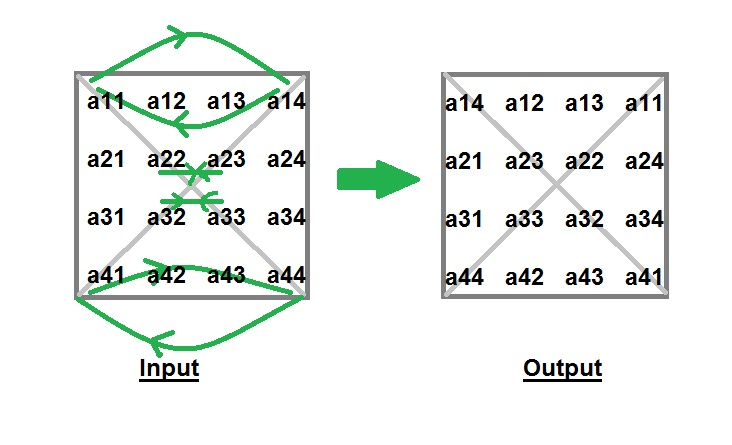GeeksforGeeks App
Open AppBrowser
Continue

# Program to Interchange Diagonals of Matrix

Given a square matrix of order n*n, you have to interchange the elements of both diagonals.

Examples :

```Input : matrix[][] = {1, 2, 3,
4, 5, 6,
7, 8, 9}
Output : matrix[][] = {3, 2, 1,
4, 5, 6,
9, 8, 7}

Input : matrix[][] = {4,  2,  3,  1,
5,  7,  6,  8,
9, 11, 10, 12,
16, 14, 15, 13}
Output : matrix[][] = {1,  2,  3,  4,
5,  6,  7,  8,
9, 10, 11, 12,
11, 14, 15, 16}```

Explanation : Idea behind interchanging diagonals of a square matrix is simple. Iterate from 0 to n-1 and for each iteration you have to swap a[i][i] and a[i][n-i-1].Implementation:

## C++

 `// C++ program to interchange``// the diagonals of matrix``#include``using` `namespace` `std;` `#define N 3` `// Function to interchange diagonals``void` `interchangeDiagonals(``int` `array[][N])``{``    ``// swap elements of diagonal``    ``for` `(``int` `i = 0; i < N; ++i)``    ``if` `(i != N / 2)``    ``swap(array[i][i], array[i][N - i - 1]);` `    ``for` `(``int` `i = 0; i < N; ++i)``    ``{``    ``for` `(``int` `j = 0; j < N; ++j)``            ``cout<<``" "``<< array[i][j];``    ``cout<

## C

 `// C program to interchange``// the diagonals of matrix``#include``using` `namespace` `std;` `#define N 3` `// Function to interchange diagonals``void` `interchangeDiagonals(``int` `array[][N])``{``    ``// swap elements of diagonal``    ``for` `(``int` `i = 0; i < N; ++i)``    ``if` `(i != N / 2)``    ``swap(array[i][i], array[i][N - i - 1]);` `    ``for` `(``int` `i = 0; i < N; ++i)``    ``{``    ``for` `(``int` `j = 0; j < N; ++j)``            ``printf``(``" %d"``, array[i][j]);``    ``printf``(``"\n"``);``    ``}``}` `// Driver Code``int` `main()``{``    ``int` `array[N][N] = {4, 5, 6,``                    ``1, 2, 3,``                    ``7, 8, 9};``    ``interchangeDiagonals(array);``    ``return` `0;``}`

## Java

 `// Java program to interchange``// the diagonals of matrix``import` `java.io.*;` `class` `GFG``{``    ``public` `static` `int` `N = ``3``;``    ` `    ``// Function to interchange diagonals``    ``static` `void` `interchangeDiagonals(``int` `array[][])``    ``{``        ``// swap elements of diagonal``        ``for` `(``int` `i = ``0``; i < N; ++i)``            ``if` `(i != N / ``2``)``            ``{``                ``int` `temp = array[i][i];``                ``array[i][i] = array[i][N - i - ``1``];``                ``array[i][N - i - ``1``] = temp;``            ``}` `        ``for` `(``int` `i = ``0``; i < N; ++i)``        ``{``            ``for` `(``int` `j = ``0``; j < N; ++j)``                ``System.out.print(array[i][j]+``" "``);``            ``System.out.println();``        ``}``    ``}``    ` `    ``// Driver Code``    ``public` `static` `void` `main (String[] args)``    ``{``        ``int` `array[][] = { {``4``, ``5``, ``6``},``                        ``{``1``, ``2``, ``3``},``                        ``{``7``, ``8``, ``9``}``                        ``};``        ``interchangeDiagonals(array);``    ``}``}` `// This code is contributed by Pramod Kumar`

## Python3

 `# Python program to interchange``# the diagonals of matrix``N ``=` `3``;` `# Function to interchange diagonals``def` `interchangeDiagonals(array):` `    ``# swap elements of diagonal``    ``for` `i ``in` `range``(N):``        ``if` `(i !``=` `N ``/` `2``):``            ``temp ``=` `array[i][i];``            ``array[i][i] ``=` `array[i][N ``-` `i ``-` `1``];``            ``array[i][N ``-` `i ``-` `1``] ``=` `temp;``        ` `    ``for` `i ``in` `range``(N):``        ``for` `j ``in` `range``(N):``            ``print``(array[i][j], end ``=` `" "``);``        ``print``();` `# Driver Code``if` `__name__ ``=``=` `'__main__'``:``    ``array ``=` `[ ``4``, ``5``, ``6` `],[ ``1``, ``2``, ``3` `],[ ``7``, ``8``, ``9` `];``    ``interchangeDiagonals(array);``    ` `# This code is contributed by Rajput-Ji`

## C#

 `// C# program to interchange``// the diagonals of matrix``using` `System;` `class` `GFG``{``    ``public` `static` `int` `N = 3;``    ` `    ``// Function to interchange diagonals``    ``static` `void` `interchangeDiagonals(``int` `[,]array)``    ``{``        ``// swap elements of diagonal``        ``for` `(``int` `i = 0; i < N; ++i)``            ``if` `(i != N / 2)``            ``{``                ``int` `temp = array[i, i];``                ``array[i, i] = array[i, N - i - 1];``                ``array[i, N - i - 1] = temp;``            ``}` `        ``for` `(``int` `i = 0; i < N; ++i)``        ``{``            ``for` `(``int` `j = 0; j < N; ++j)``                ``Console.Write(array[i, j]+``" "``);``            ``Console.WriteLine();``        ``}``    ``}``    ` `    ``// Driver Code``    ``public` `static` `void` `Main ()``    ``{``        ``int` `[,]array = { {4, 5, 6},``                        ``{1, 2, 3},``                        ``{7, 8, 9}``                        ``};``        ``interchangeDiagonals(array);``    ``}``}` `// This code is contributed by vt_m.`

## Javascript

 ``

Output

``` 6 5 4
1 2 3
9 8 7```

Time Complexity: O(N*N), as we are using nested loops for traversing the matrix.
Auxiliary Space: O(1), as we are not using any extra space.